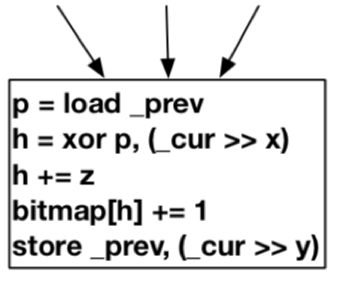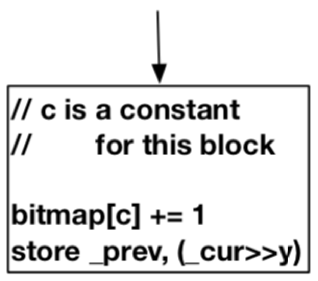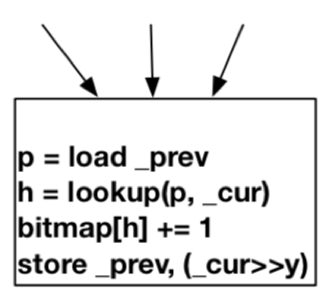# 0x00 — Begin

Authors

Shuitao Gan, State Key Laboratory of Mathematical Engineering and Advanced Computing

Chao Zhang, Tsinghua University

Xiaojun Qin, State Key Laboratory of Mathematical Engineering and Advanced Computing

Xuwen Tu, State Key Laboratory of Mathematical Engineering and Advanced Computing

Kang Li, Cyber Immunity Lab

Zhongyu Pei, Tsinghua University

Zuoning Chen, National Research Center of Parallel Computer Engineering and Technology

# 0x03 How

CollAFL的具体做法如下。

cur ⊕ (prev ≫ 1)F mul(cur, prev) = (cur ≫ x) ⊕ (prev ≫ y) + z

具有Single Precedent块的hash算法F single(cur, prev) : c

具有Multiple Precedents块的hash算法F hash(cur, prev) : hash_table_lookup(cur, prev)

整体缓解方案


\begin{cases} F mul,\\ F hash\\ F single \end{cases}

CollAFL-br

Weight_Br(T) =\sum_{bb∈P ath(T )<bb,bb_i>∈EDGES}IsUntouched(< bb,bb_i >)

CollAFL-desc

Weight_Desc(T) = \sum_{bb∈P ath(T )IsU ntouched(<bb,bb_i >)}NumDesc(bb_i)

NumDesc(bb) =\sum_{<bb,bb_i>∈EDGES}NumDesc(bb_i)

CollAFL-mem

Weight_Mem(T) =\sum_{bb∈P ath(T )}NumMemInstr(bb)

# 0x04 Result

• 在24个实际应用程序中发现了157个新的安全漏洞，其中95个被CVE确认。
• 没有开源CollAFL